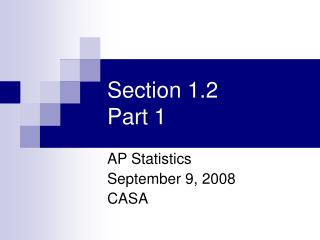DownloadDownload PresentationSection 1.2 Part 1

# Section 1.2 Part 1

Télécharger la présentation## Section 1.2 Part 1

- - - - - - - - - - - - - - - - - - - - - - - - - - - E N D - - - - - - - - - - - - - - - - - - - - - - - - - - -
##### Presentation Transcript

1. Section 1.2Part 1 AP Statistics September 9, 2008 CASA

2. Describing Distributions with Numbers: Center/Mean • The Mean • The Average • The Arithmetical Mean • The mean is not a resistance measure of center AP Statistics, Section 1.2, Part 1

3. Describing Distributions with Numbers: Center/Median • When numbers are ordered from low to high, the median is the middle number (if n is odd) or the average of the two middle numbers (if n is even) • Resistant measure of center AP Statistics, Section 1.2, Part 1

4. Describing Distributions with Numbers: Spread/IQR • The first quartile (Q1) is the median of the first half of the distribution • The third quartile (Q3) is the median of the second half of the distribution • The interquartile range (IQR) is the distance between the first and third quartiles. (IQR = Q3 – Q1) AP Statistics, Section 1.2, Part 1

5. Finding M, Q1,Q3,IQR • Barry Bonds’ home run counts per season (average=35.4):16, 19, 24, 25, 25, 33, 33, 34, 34, 37, 37, 40, 42, 46, 49, 73 • Hank Aaron’s home run counts per season (average=34.9):13, 20, 24, 26, 27, 29, 30, 32, 34, 34, 38, 39, 39, 40, 40, 44, 44, 44, 44, 45, 47 AP Statistics, Section 1.2, Part 1

6. Finding M, Q1,Q3,IQR • Barry Bonds: Q1=25,M=34, Q3=41,IQR=16 • Hank Aaron: Q1=28,M=38, Q3=44,IQR=16 • What does this say about comparing Bonds and Aaron? AP Statistics, Section 1.2, Part 1

7. Describing Distributions with Numbers: Outliers • If a data point is greater than Q3 +1.5 * IQR • or • If a data point is less than Q1 - 1.5 * IQR . • Barry Bonds: M=34, Q1=25,Q3=41,IQR=16 • 41 + 1.5 * 16 = 65 (anything above 65) • 25 - 1.5 * 16 = 1 (anything below 1) AP Statistics, Section 1.2, Part 1

8. Describing Distributions with Numbers: 5 Number Summary • We can summarize a distribution (a data set) with 5 numbers • Minimum • Q1 • Median • Q3 • Maximum AP Statistics, Section 1.2, Part 1

9. Boxplot AP Statistics, Section 1.2, Part 1

10. Modified Boxplot AP Statistics, Section 1.2, Part 1

11. Assignment • Exercises 1.31 – 1.39, The Practice of Statistics. AP Statistics, Section 1.2, Part 1